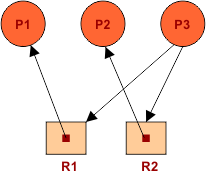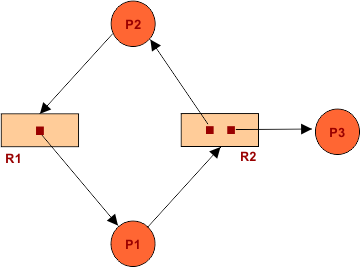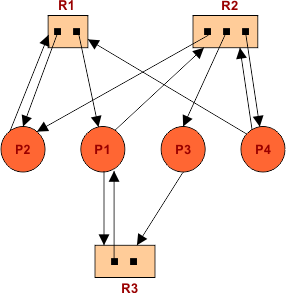Select Page

In the last lecture, we have cover Deadlock Prevention and Avoidance. In this lecture, we will see Deadlock Detection.

Deadlock detection can be explained in two terms:

```1. When resources have single instance
2. And When resources have multiple instances

```

So, To cover Deadlock detection we first have to understand the concept of resource allocation graph. Because Deadlock can be detect through resource allocation graph.

## What Is a Resource Allocation Graph?

The resource allocation graph provides the entire information about all those processes which are holding some resources or waiting for resources.  If there is a single instance resource in the cycle and circular wait exist then deadlock must be there.

Any resource in the cycle may have more than one instance. Each instance of resource in cycle is represented by a dot inside the rectangle.

## How Wait for Graph is Derived?

P1 will complete when it will get resources R1 and R2 given is resource allocation Graph. R1 is already allocated to P1 but R2 is given to P2. So P1 is dependent on P2, show in wait for graph P1àP2. Because when P2 executed then it will free the allocated resource R2. In the same way P2 will get executed when it will get resources R3, R4 and R5. R3 is taken by P5, R4 is taken by P3 and R5 is taken by P3. So, P2 is dependent on P3, P4 and P5. In the same way P3 is dependent on P4. Because P4 reserved the resources required to P3. P1 reserved the resources required to P4 so, P4 is dependent on P1.

## Resource Allocation Graph Vs Wait for Graph

If the is a cycle in wait for graph then there must be deadlock but if there is cycle in resource allocation graph then there may or may not be a deadlock.

## Components of Resource Allocation Graph

There are two main components of resource allocation graph

``` i. Vertices
The sub-components of Vertices are Process vertex and Resource Vertex. The resource vertex
is further categorized into Single instance Resource and multi instance resource.```

```ii. Edge
Edge is also known as arrow. Edge is further categorized into Reguset edge and assign edge
detail diagram of components of resource allocation graph is given under```

### Through Resource allocation graph we can find the followings:

• Sequence?
• In the end current available resources?

Now let explain the deadlock detection through its methods:

### 1. When Resources Have Single Instance

If CPU is the Resource and our system having only one CPU, then it will be single instance Resource. In the same way, If Printer is the resource and we have only one printer, then it is an example of single instance resource.

Keep in mind, If there is a cycle in resource allocation graph and all resources are single instance in that cycle then there must be a deadlock in processes.

### Question 01: Look at Resource allocation graph given below and find weather a deadlock present or not?

To find the deadlock in resource allocation graph there is a best way called resource allocation table. Explanation is given under.

So, Deadlock is confirmed because P1 is dependent on P2 and vice versa. But not resource is available. There were two resources and both of these are used.

Question No: 02

Look at Resource allocation graph in the diagram. And find whether the deadlock is there or not? and if there is no deadlock then find the safe sequence?Solution:

Safe Sequence will be P1 -> P2 -> P3. No deadlock because all processes executed successfully.

Note: Availability (0, 0) can be check from diagram as two resources and taken by some processes. When one process execute then its resources are taken back and availability of resources increased.

## 2. When resources have multiple instances

### Question No: 01

Find whether the deadlock is there or not? and if there is no deadlock then find the safe sequence? Resource allocation graph for this question is given belowSafe Sequence will be P3 -> P1 -> P2. No deadlock because all processes executed successfully.

### Question No: 02

Resource allocation graph is given in diagram. Find deadlock is there or not? and if there is no deadlock then find the safe sequence?The safe sequence will be P3 -> P1 -> P2 -> P4. After execution all resources will get free.

Note:If a single instance resource and circular wait exist then deadlock must be there. If a multi instance resource and circular wait exist then deadlock may or may not be there

For example, If register is a resource then Register R1,R2,R3….Rn are the instances of this resource.

Help Other’s By Sharing…